Fluid

General information

Newtonian fluid

A fluid in which the components of the stress tensor are linear functions

of the first spatial derivatives of the velocity components. These functions

involve two material parameters (taken as constants throughout the fluid,

although depending on ambient temperature and pressure).

1979, 51, 1216

Reference: IUPAC Compendium of Chemical Terminology 2nd Edition (1997)

Non-Newtonian Fluid-shear dependent viscosity

For systems showing non-Newtonian behaviour when measured in steady

simple shear, a coefficient h equal to s ¤ D at a given value of the shear

rate D, where s is the stress; h0 is the limiting viscosity at zero shear rate,

and h¥ the limiting viscosity at infinite shear rate; [ h0] is the limit of

intrinsic viscosity at zero shear.

1979, 51, 1217

Newtonian fluid

A fluid in which the components of the stress tensor are linear functions

of the first spatial derivatives of the velocity components. These functions

involve two material parameters (taken as constants throughout the fluid,

although depending on ambient temperature and pressure).

1979, 51, 1216

Dynamic viscosity, h

For a laminar flow of a fluid the ratio of the shear stress to the velocity

gradient perpendicular to the plane of shear.

G.B. 13

shear stress, t

Force acting tangentially to a surface divided by the area of the surface.

G.B. 12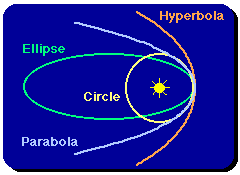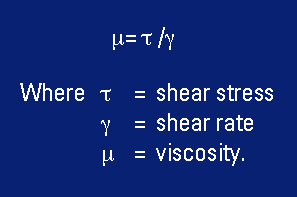Simple shear

An idealized treatment of a fluid between two large parallel plates (to

permit ignoring edge effects) of area A, separated by a distance h. If one

plate moves relative to the other with a constant velocity V, requiring a

force F acting in the direction of movement, and the density, pressure, and

viscosity throughout the fluid are constant, the Newtonian equation can be

coupled with the equations of motion and of continuity to show that the

velocity gradient in the fluid is constant (= V/h), and that F/A = hV ¤ h This

idealized case (simple shear) is sometimes used to define shear viscosity.

1979, 51, 1216

shear rate

The velocity gradient in a flowing fluid.

1992, 64, 165

Reduced viscosity (of a polymer)

The ratio of the relative viscosity increment to the mass concentration of

the polymer, c, i.e. hi ¤ c, where hi is the relative viscosity increment.

Notes:

1. The unit must be specified; cm3 g–1 is recommended.

2. This quantity is neither a viscosity nor a pure number. The term is to be

looked on as a traditional name. Any replacement by consistent terminology

would produce unnecessary confusion in the polymer literature.

Synonymous with viscosity number.

P.B. 63

Apparent viscosity (of a liquid)

The ratio of stress to rate of strain, calculated from measurements of forces

and velocities as though the liquid were Newtonian. If the liquid is actually

non-Newtonian, the apparent viscosity depends on the type and dimensions

of the apparatus used.

1979, 51, 1217

Polymer dynamics and rheologyPolymers are long molecules made from joining together lots of small molecules (or monomers). Sometimes polymer molecules are linear, but very often - notably in the case of Low Density Polyethylene (LDPE) used to make plastic bottles - they include many branches.

During the manufacture of polymeric (or plastic) materials and commodities, liquids containing polymers are subjected to flow. The way these liquids react is determined by the shapes, or configurations that the molecules adopt. Polymer molecules behave like springs, and become stretched by the flow, giving rise to the strongly elastic behaviour of polymeric fluids. The study of the dynamics of polymer molecules is very important for the understanding of flow of polymeric fluids.

If polymer molecules overlap sufficiently, then they get tangled up (like spaghetti) so that they are constrained in their movement. The "tube model" for entangled polymers provides a conceptual framework for understanding the constrained motion, and for making mathematical predictions about the polymers' response to flow.

Branch points in the polymer molecules provide additional obstacles to the motion of entangled polymers, so that the distribution of branch points in polymer molecules can be a critical factor in determining flow properties.

## Non-Newtonian fluidsElastic stresses in polymeric and other complex fluids can give rise to strange flow behaviour not seen in Newtonian fluids. This can, for example, produce undesirable instabilities in industrial processes. The aim of our research is to predict how these fluids will flow in various flow geometries and to determine the conditions for the flow to become unstable. Our current research includes investigations of how bubbles grow in polyurethane foams; how filaments of polymer stretch and break-up; and flow instabilities in extrusion.

## Reaction chemistry and branched polymer architectureThere are different chemical routes used to produce branched polymers in an industrial setting. The particular reaction chemistry, and the reactor type and conditions, have a large effect on the number and distribution of branches throughout the polymer molecules. Current research is examining this relationship between reaction chemistry and branched polymer architecture with a view towards the reaction design for polymer melts with tailored flow properties.

## Polymer dynamics and neutron scatteringIt is important to understand the shapes, or conformations that polymers take under flow conditions. Although polymer rheology (the stress response of the fluid) is one way of probing this, it is important to have other independent tools to check that the theory is right. A more direct measure of polymer shape is obtained via neutron scattering from polymers that are partially labeled with deuterium. We aim to predict neutron scattering patterns from deformed polymer melts.

## Suspension mechanics

Another important class of non-Newtonian fluids is suspensions of solid particles, such as spheres and fibers, or droplets or bubbles in Newtonian or non-Newtonian fluids. For example, short glass and carbon fibers are often added to injection molded plastics to reduce cost and to improve the mechanical or thermal properties of the finished product. Small spheres are often suspended in a fluid in order to transport, for example, pharmaceutical powders around processing plants; and many food-stuffs and skin-care products are formed from oil-in-water emulsions. While the motion of single particles in Newtonian fluids is well understood, suspensions of large numbers of particles that interact through the fluid remain challenging, both analytically and numerically. Our current research includes investigations of how rough particle surfaces can affect the flow of a suspension of solid spheres; how collisions between fibers affect the flow properties of concentrated fiber suspensions; propagation of sound waves through colloidal suspensions; and how droplets deform in a polymeric fluid flow.

Non-Newtonian Flows

The viscosity of Newtonian fluids such as air and water is independent of the shear rate, while non-Newtonian fluids exhibit viscosity characteristics that are a function of shear rate. Non-Newtonian effects can play a dramatic role in modeling already complex problems such as multi-phase separation due to the changing shear the fluid experiences as it progresses through the system.

Rather than modeling strictly Newtonian fluids, we have developed extensive expertise using products such as carboxymethyl-cellulose (CMC) to model industrial non-Newtonian fluids. Along with our high-viscosity non-Newtonian capability, we also have experience modeling foaming phenomena and elastic properties of fluids. In addition to mixing and pumping equipment for these fluids, we have a Brookfield LVDVIII Rheometer to characterize the fluid properties.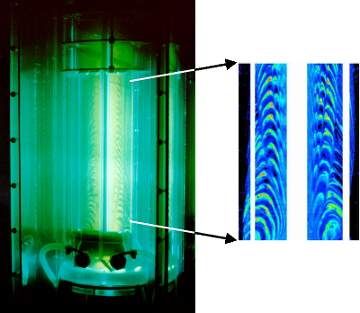Mixing study -bulk fluid was high viscosity and shear-thinning, and the injected fluid was plain water. The image to the right is a 2D LIF image of the concentration of the injected fluid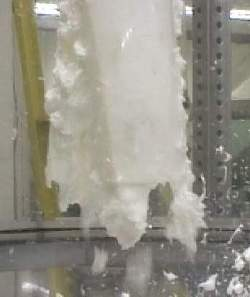A non-Newtonian, elastic, foaming fluid  to model a polymer melt undergoing a pressure letdown.

References:

http://www.maths.leeds.ac.uk/Applied/index.html

http://www.coanda.ca/non-Newtonian.html

IUPAC Compendium of Chemical Terminology 2nd Edition (1997)

http://www.iupac.org/index_to.html

Non-Newtonian Flows

Fluids such as water, air, ethanol, and benzene are Newtonian. This means that a plot of shear stress versus shear rate at a given temperature is a straight line with a constant slope that is independent of the shear rate. We call this slope the viscosity of the fluid. All gases are Newtonian. Also, low molecular weight liquids, and solutions of low molecular weight substances in liquids are usually Newtonian. Some examples are aqueous solutions of sugar or salt. Any fluid that does not obey the Newtonian relationship between the shear stress and shear rate is called non-Newtonian. The subject of “Rheology” is devoted to the study of the behavior of such fluids. High molecular weight liquids which include polymer melts and solutions of polymers, as well as liquids in which fine particles are suspended (slurries and pastes), are usually non-Newtonian. In this case, the slope of the shear stress versus shear rate curve will not be constant as we change the shear rate. When the viscosity decreases with increasing shear rate, we call the fluid shear-thinning. In the opposite case where the viscosity increases as the fluid is subjected to a higher shear rate, the fluid is called shear-thickening. Shear-thinning behavior is more common than shear-thickening. Shear-thinning fluids also are called pseudoplastic fluids. A typical shear stress versus shear rate plot for a shear-thinning fluid looks like this.We describe the relationship between the shear stress τ and shear rate θ? as follows.

T=η.(θ)'

Here, η is called the “apparent viscosity” of the fluid, and is a function of the shear rate. In the above example, a plot of η as a function of the shear rate (θ)' looks like this.Many shear-thinning fluids will exhibit Newtonian behavior at extreme shear rates, both low and high. For such fluids, when the apparent viscosity is plotted against log shear rate, we see a curve like this.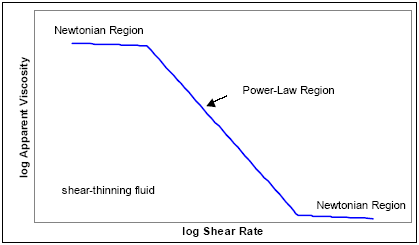The regions where the apparent viscosity is approximately constant are known

as Newtonian regions. The behavior between these regions can usually be

approximated by a straight line on these axes. It is known as the power-law

region. In this region, we can approximate the behavior by

log (η)=a + b log (θ)' which can be rewritten as

η=K((θ)')^b=? where K=exp (a) . Instead of b we commonly use (n1) for the exponent and write a result for the apparent viscosity as follows.

η=K ((θ)'^(n-1)

Upon using the connection among the shear stress, apparent viscosity, and the shear rate we get the power-law model.

τ=K ((θ)')^n where n is called the power-law index. Note that n=1

corresponds to Newtonian behavior. Typically, for shear thinning fluids, n lies between 1/3 and 1/2, even though other values are possible.

Examples of shear-thinning fluids are polymer melts such as molten polystyrene, polymer solutions such as polyethylene oxide in water, and some paints. You can see that when paint is sheared with a brush, it flows comfortably, but when the shear stress is removed, its viscosity increases so that it no longer flows easily. Of course, the solvent evaporates soon and then the paint sticks to the surface. The behavior of paint is a bit more complex than this, because the viscosity changes with time at a given  shear rate.

Some slurries and pastes exhibit an increase in apparent viscosity as the shear rate is increased. They are called shear-thickening or dilatants fluids. Typical plots of shear stress versus shear rate and apparent viscosity versus shear rate  are shown next.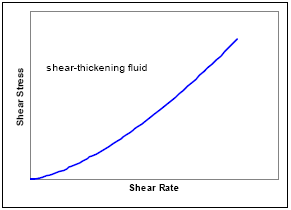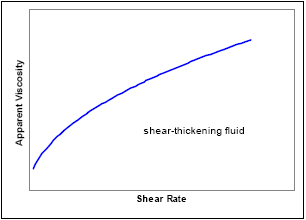Some examples of shear-thickening fluids are corn starch, clay slurries, and solutions of certain surfactants. Most shear-thickening fluids tend to show shear-thinning at very low shear rates.

Another important type of non-Newtonian fluid is a visco-plastic or “yield stress” fluid. This is a fluid which will not flow when only a small shear stress is applied. The shear stress must exceed a critical value known as the yield stress T0

for the fluid to flow.

For example, when you open a tube of toothpaste, it would be good if the paste does not flow at the slightest amount of shear stress. We need to apply an adequate force before the toothpaste will start flowing. So, visco-plastic fluids behave like solids when the applied shear stress is less than the yield stress. Once it exceeds the yield stress, the visco-plastic fluid will flow just like a fluid.

Bingham plastics are a special class of visco-plastic fluids that exhibit a linear behavior of shear stress against shear rate. Typical visco-plastic behaviors are illustrated in the next figure.Examples of visco-plastic fluids are drilling mud, nuclear fuel slurries, mayonnaise, toothpaste, and blood. Also, some paints exhibit a yield stress.

Of course, this is not an exhaustive discussion of non-Newtonian behaviors. For instance, some classes of fluids exhibit time-dependent behavior. This means that even under a given constant shear rate, the viscosity may vary with time. The viscosity of a thixotropic liquid will decrease with time under a constant applied shear stress. However, when the stress is removed, the viscosity will gradually recover with time as well. Non-drip paints behave in this way.

The opposite behavior, wherein the fluid increases in viscosity with time when a constant shear stress is applied is not as common, and such a fluid is called a rheopectic fluid.

Another important class of fluids exhibits visco-elastic behavior. This means that these fluids behave both as solids (elastic) and fluids (viscous). visco-elastic fluids exhibit strange phenomena such as climbing up a rotating shaft, swelling extruded out of a dye, etc. An example of common visco-elastic liquid is egg-white. you have probably noticed that when it flows out of a container, you can use a quick jeking motion to snap is back into the container. several industrially important polymer melts and solutions are visco-elastic. you can learn more about these and other behaviors from several fluid mechanics textbooks and from Perry’s Chemical Engineers’ Handbook.